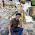### Class X Maths

Syllabus

___________________________________________________________________________________

28/04/2017 -- 10th C

Holiday Home Work Class X Maths

PROJECT WORK ( Choose any One) Do in activity book

1.Make a PPT  on any lesson

2.Write a problem on application of linear equations in 2 variables and solve
graphically(See text book  chapter 3) Do in activity book

3. Collect information on Origin and development of number system. Do in activity book

Solve the following problems in home work book

1 State Euclids  Division Lemma

2.State fundamental theorem of arithmetic

3. Find HCF using Euclid’s division algorithm 804, 4355

4. Prove that √3 is irrational

5. Prove that 2+3√5 is irrational

6. State whether the following have terminating decimal representation or not:13/625      1/7

7. Find zeroes of the following polynomials and verify the relationship between its coefficients: i) 3 x2  −5 x−28

8. If √7, -√7 are zeroes of 2 x4  +3 x3  −16 x2−21 x+14 , find the other two zeroes

9. Find the zeroes of polynomial x3 −2 x2− x+2

10. Solve graphically: 3x +y +1=0, 2x -3y +8=0

1.